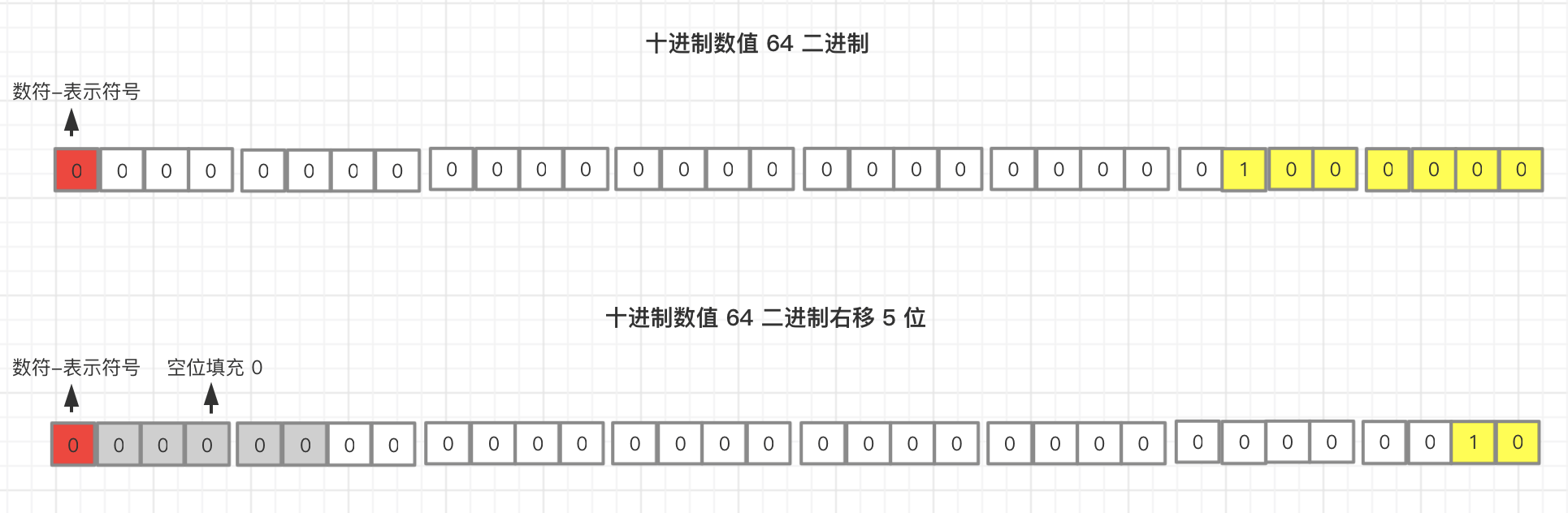星期二, 十二月 1日 2020, 8:00 早上

5.2k 字     19 分钟

# 「硬核 JS」令你迷惑的位运算

## 写在前面

``(!(~+[])+{})[--[~+""][+[]]*[~+[]]+~~!+[]]+({}+[])[[~!+[]]*~+[]]``

## 位运算

### 按位非 NOT（~）

#### 简述

``````let a = 1
console.log(~a == (-a) - 1) // true

// 得到
~x = (-x) - 1``````

#### 使用按位非 ~ 判断是否等于-1

``````let str = "abcdefg"

if(!~str.indexOf("n")){
console.log("字符串 str 中不存在字符 n")
}

// 等同于

if(str.indexOf("n") == "-1"){
console.log("字符串 str 中不存在字符 n")
}``````

#### 使用按位非 ~ 取整

``~~3.14 == 3``

``````~3.14 == ~3
-5.89 == ~5``````

``````~x == (-x) - 1

~~x == -((-x) - 1) -1 == -(-x) + 1 -1 == x``````

### 按位与 AND（&）

#### 简述`25 & 3` ，即求十进制 25 和 十进制 3 的与操作值

``````25 = 0000 0000 0000 0000 0000 0000 0001 1001
3 = 0000 0000 0000 0000 0000 0000 0000 0011
--------------------------------------------
&  = 0000 0000 0000 0000 0000 0000 0000 0001``````

#### 使用按位与 & 判断奇偶数

``````偶数 & 1 // 0

#### 使用按位与 & 判断数字是否为2的整数幂

``````let a = 20;
let b = 32;

a & (a - 1) // 16 a不是2的整数幂
b & (b - 1) // 0     b是2的整数幂``````

``````0000 0001  -> 1      // 2^0
0000 0010  -> 2        // 2^1
0000 0100  -> 4        // 2^2
0000 1000  -> 8        // 2^3
0001 0000  -> 16    // 2^4``````

### 按位或 OR（｜）

#### 简述`25 ｜ 3` ，即求十进制 25 和 十进制 3 的或操作值

``````25 = 0000 0000 0000 0000 0000 0000 0001 1001
3 = 0000 0000 0000 0000 0000 0000 0000 0011
--------------------------------------------
|  = 0000 0000 0000 0000 0000 0000 0001 1011``````

#### 使用按位或 ｜ 取整

``````1.111 | 0 // 1
2.234 | 0 // 2``````

0 的二进制全是 0 ，按位或对比时 1 和 0 就是 1 ，0 和 0 就是 0，得出的二进制就是我们要取整数值的整数部分

#### 使用按位或 | 代替Math.round()

``````let a1 = 1.1
let a2 = 1.6
a1 + 0.5 | 0 // 1
a2 + 0.5 | 0 // 2

let b1 = -1.1
let b2 = -1.6
b1 - 0.5 | 0 // -1
b2 - 0.5 | 0 // -2``````

### 按位异或 XOR（^）

#### 简述`25 ^ 3` ，即求十进制 25 和 十进制 3 的异或值

``````25 = 0000 0000 0000 0000 0000 0000 0001 1001
3 = 0000 0000 0000 0000 0000 0000 0000 0011
--------------------------------------------
|  = 0000 0000 0000 0000 0000 0000 0001 1010``````

#### 使用按位异或 ^ 判断整数部分是否相等

``````let a = 1
let b = 1
a ^ b // 0

1 ^ 1 // 0
2 ^ 2 // 0
3 ^ 3 // 0``````

``````2.1 ^ 2.5 // 0
2.2 ^ 2.6 // 0
2.1 ^ 3.1 // 1``````

``````2 ^ 2 // 0
2 ^ 2 // 0
2 ^ 3 // 1``````

#### 使用按位异或 ^ 来完成值交换

``````let a = 1
let b = 2
a ^= b
b ^= a
a ^= b
console.log(a)   // 2
console.log(b)   // 1``````

``````// 如果
a ^ b = c
// 那么
c ^ b = a
c ^ a = b``````

#### 使用按位异或 ^ 切换 0 和 1

``````let toggle = 0

if(toggle){
toggle = 0
}else{
toggle = 1
}``````

``````let toggle = 0

toggle = toggle ? 0 : 1``````

``````let toggle = 0

toggle ^= 1``````

#### 使用按位异或 ^ 判断两数符号是否相同

``````let a = 1
let b = 2
let c = -2

(a ^ b) >= 0 // true
(a ^ c) >= 0 // false``````

### 左移（<<）

#### 简述

*例如： *

`2 << 5`，即求十进制数 2 左移 5 位的操作``````x << y

// 等同于

x * 2^y``````

#### 使用左移 << 取整

``````1.111 << 0 // 1
2.344 << 0 // 2``````

### 有符号右移（>>）

#### 简述

`64 >> 5`，即求十进制数 64 有符号右移 5 位的操作``````x >> y

// 等同于

x / 2^y``````

#### 使用右移 >> 取整

``````1.111 >> 0 // 1
2.344 >> 0 // 2``````

### 无符号右移（>>>）

#### 简述

-1 是负数，在内存中二进制存储是补码即 `1111 .... 1111 1111`，32 位都是 1，我们在程序中写入 `-1 >>> 0` 运行得到十进制数字 `4294967295` ，再使用二进制转换工具转为二进制得到的就是 32 位二进制 `1111 .... 1111 1111`，所以就算无符号右移 0 位，得出的依然是一个很大的正数

#### 使用无符号右移 >>> 取整（正数）

``````1.323 >>> 0 // 1
2.324 >>> 0 // 2``````

## 最后